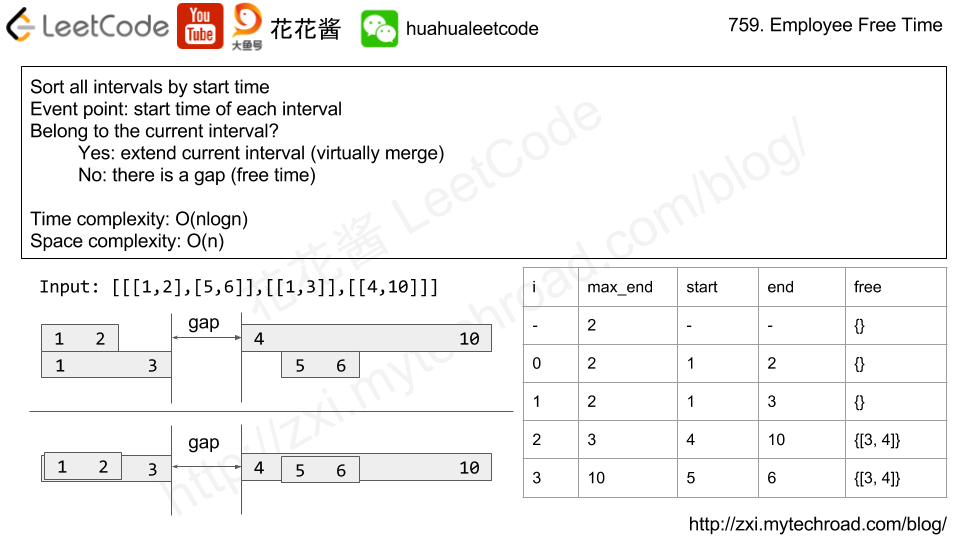# Posts tagged as “interval”

Given two integer arrays startTime and endTime and given an integer queryTime.

The ith student started doing their homework at the time startTime[i] and finished it at time endTime[i].

Return the number of students doing their homework at time queryTime. More formally, return the number of students where queryTime lays in the interval [startTime[i], endTime[i]] inclusive.

Example 1:

Input: startTime = [1,2,3], endTime = [3,2,7], queryTime = 4
Output: 1
Explanation: We have 3 students where:
The first student started doing homework at time 1 and finished at time 3 and wasn't doing anything at time 4.
The second student started doing homework at time 2 and finished at time 2 and also wasn't doing anything at time 4.
The third student started doing homework at time 3 and finished at time 7 and was the only student doing homework at time 4.


Example 2:

Input: startTime = , endTime = , queryTime = 4
Output: 1
Explanation: The only student was doing their homework at the queryTime.


Example 3:

Input: startTime = , endTime = , queryTime = 5
Output: 0


Example 4:

Input: startTime = [1,1,1,1], endTime = [1,3,2,4], queryTime = 7
Output: 0


Example 5:

Input: startTime = [9,8,7,6,5,4,3,2,1], endTime = [10,10,10,10,10,10,10,10,10], queryTime = 5
Output: 5


Constraints:

• startTime.length == endTime.length
• 1 <= startTime.length <= 100
• 1 <= startTime[i] <= endTime[i] <= 1000
• 1 <= queryTime <= 1000

## Solution: Brute Force

Time complexity: O(n)
Space complexity: O(1)

## Python3

Implement the class TweetCounts that supports two methods:

1. recordTweet(string tweetName, int time)

• Stores the tweetName at the recorded time (in seconds).

2. getTweetCountsPerFrequency(string freq, string tweetName, int startTime, int endTime)

• Returns the total number of occurrences for the given tweetName per minutehour, or day (depending on freq) starting from the startTime (in seconds) and ending at the endTime (in seconds).
• freq is always minutehour or day, representing the time interval to get the total number of occurrences for the given tweetName.
• The first time interval always starts from the startTime, so the time intervals are [startTime, startTime + delta*1>,  [startTime + delta*1, startTime + delta*2>, [startTime + delta*2, startTime + delta*3>, ... , [startTime + delta*i, min(startTime + delta*(i+1), endTime + 1)> for some non-negative number i and delta (which depends on freq).

Example:

Input
["TweetCounts","recordTweet","recordTweet","recordTweet","getTweetCountsPerFrequency","getTweetCountsPerFrequency","recordTweet","getTweetCountsPerFrequency"]
[[],["tweet3",0],["tweet3",60],["tweet3",10],["minute","tweet3",0,59],["minute","tweet3",0,60],["tweet3",120],["hour","tweet3",0,210]]

Output
[null,null,null,null,
[2,1],null,]

Explanation
TweetCounts tweetCounts = new TweetCounts();
tweetCounts.recordTweet("tweet3", 0);
tweetCounts.recordTweet("tweet3", 60);
tweetCounts.recordTweet("tweet3", 10);                             // All tweets correspond to "tweet3" with recorded times at 0, 10 and 60.
tweetCounts.getTweetCountsPerFrequency("minute", "tweet3", 0, 59); // return . The frequency is per minute (60 seconds), so there is one interval of time: 1) [0, 60> - > 2 tweets.
tweetCounts.getTweetCountsPerFrequency("minute", "tweet3", 0, 60); // return [2, 1]. The frequency is per minute (60 seconds), so there are two intervals of time: 1) [0, 60> - > 2 tweets, and 2) [60,61> - > 1 tweet.
tweetCounts.recordTweet("tweet3", 120);                            // All tweets correspond to "tweet3" with recorded times at 0, 10, 60 and 120.
tweetCounts.getTweetCountsPerFrequency("hour", "tweet3", 0, 210);  // return . The frequency is per hour (3600 seconds), so there is one interval of time: 1) [0, 211> - > 4 tweets.



Constraints:

• There will be at most 10000 operations considering both recordTweet and getTweetCountsPerFrequency.
• 0 <= time, startTime, endTime <= 10^9

## Solution: Hashtable + binary search

Time complexity:
Record: O(logn)
getCount: O(logn + |entries|)

Space complexity: O(n)

## C++

Given a sorted list of disjoint intervals, each interval intervals[i] = [a, b] represents the set of real numbers x such that a <= x < b.

We remove the intersections between any interval in intervals and the interval toBeRemoved.

Return a sorted list of intervals after all such removals.

Example 1:

Input: intervals = [[0,2],[3,4],[5,7]], toBeRemoved = [1,6]
Output: [[0,1],[6,7]]


Example 2:

Input: intervals = [[0,5]], toBeRemoved = [2,3]
Output: [[0,2],[3,5]]


Constraints:

• 1 <= intervals.length <= 10^4
• -10^9 <= intervals[i] < intervals[i] <= 10^9

## Solution: Geometry

Time complexity: O(n)
Space complexity: O(n)

## C++

Given two lists of closed intervals, each list of intervals is pairwise disjoint and in sorted order.

Return the intersection of these two interval lists.

(Formally, a closed interval [a, b] (with a <= b) denotes the set of real numbers x with a <= x <= b.  The intersection of two closed intervals is a set of real numbers that is either empty, or can be represented as a closed interval.  For example, the intersection of [1, 3] and [2, 4] is [2, 3].)

Example 1:

Input: A = [[0,2],[5,10],[13,23],[24,25]], B = [[1,5],[8,12],[15,24],[25,26]]
Output: [[1,2],[5,5],[8,10],[15,23],[24,24],[25,25]]
Reminder: The inputs and the desired output are lists of Interval objects, and not arrays or lists.


Note:

1. 0 <= A.length < 1000
2. 0 <= B.length < 1000
3. 0 <= A[i].start, A[i].end, B[i].start, B[i].end < 10^9

## Solution: Two pointers

Time complexity: O(m + n)
Space complexity: O(1)

## Python3

Problem:

We are given a list schedule of employees, which represents the working time for each employee.

Each employee has a list of non-overlapping Intervals, and these intervals are in sorted order.

Return the list of finite intervals representing common, positive-length free time for all employees, also in sorted order.

Example 1:

Example 2:

(Even though we are representing Intervals in the form [x, y], the objects inside are Intervals, not lists or arrays. For example, schedule.start = 1, schedule.end = 2, and schedule is not defined.)

Also, we wouldn’t include intervals like [5, 5] in our answer, as they have zero length.

Note:

1. schedule and schedule[i] are lists with lengths in range [1, 50].
2. 0 <= schedule[i].start < schedule[i].end <= 10^8.

Idea:

Merge Intervals (virtually)Solution:

C++

Time complexity: O(nlogn)

Space complexity: O(n)

n is the total number of intervals, n <= 2500

Related Problems:

Mission News Theme by Compete Themes.